RECEIVING LOOP AERIALS FOR 1.8 MHz

by Lloyd Butler VK5BR

Originally Published in Amateur Radio September 1990)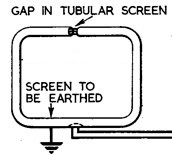Basic Shielded Loop Localised noise in the receiver can be reduced by using a small loop aerial. Shielded coax loops, unshielded loops and ferrite rod loops for 1.8 Mhz have been compared here. Best peformance has been achieved with with 6 & 1/2 turns unshielded on a 0.8 metre frame and connected via a balanced interface amplifier.

Introduction

The theory of how a small loop aerial can reduce locally generated noise is described in a previous article.

.

In the March 1982 issue of 'Amateur Radio', Clarrie Castle VK5KL described a receiving loop aerial for 1.8 MHz. The octagonal shaped loop, some three metres in length and breadth, was formed by a single turn of coaxial cable, the outer braid of which provided the electrostatic shield. From all accounts, the aerial was very successful in improving the received signal-tonoise ratio in the presence of localised noise interference.

It seemed to me that perhaps the same performance could be achieved with a loop of smaller dimensions but with more than one coaxial turn. This would allow operation in a more confined space and even inside the radio shack. With this in mind, the performance at 1.8 MHz of an 0.8m square multi-turn coax loop aerial has been investigated. Also examined is an unshielded version of the same sized loop aerial and a ferrite core loop aerial made for the 1.8MHz band. The performance of each is individually discussed and then compared.

Loop Sensitivity

For a tuned loop oriented to give maximum signal (that is, its plane in line with direction of signal source) the loop sensitivity (Es/e) can be defined as follows:

Es/e = (2nNAQ)/lambda

where Es = Output Voltage from loop
e = Field strength in Volts per metre
N = Number of loop turns
A = Loop area in square metres
Q = Loop Q factor
lambda = Wavelength in metres

Three-Turn Loop

Comparing the 0.8m square loop to the larger VK5KI, loop, the area is only 0.64 square metre compared to 6.2 square metres for the latter.This reduction factor of around 1:10 means a loop sensitivity loss of around 1:10, but this can be partly compensated by increasing the number of turns. However, increasing the number of turns also increases the inductance of the loop and its inherent shunt capacitance thus reducing the loop natural resonant frequency. This frequency must be higher than the operating frequency (1.8 MHz) otherwise it cannot be tuned to the operating frequency.

Three coax turns of 0.8 metre square appeared to approach this limit, and an experimental 0.8m square loop was assembled with three turns of 75 0hm TV coax. There was no particular reason for selecting this type of coax except that I happened to have a piece just the right length! The construction of this aerial is illustrated in figures 1 and 2. Observe that the outer braids of each of the coax turns are broken at the apex of the loop, and all braids are joined at the base of the loop. The square loop is oriented with its diagonals vertical and horizontal. The reason for this is that it is convenient to mount the loop interface box, with its connection to the loop, on one of the crossed pieces of wood which support the loop. It also makes it convenient to hang the loop from a hook in the wood at the apex.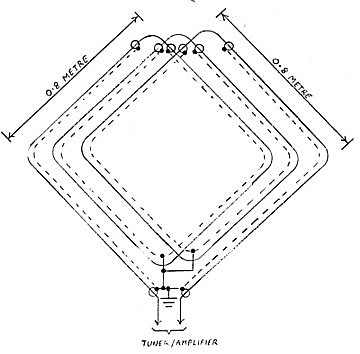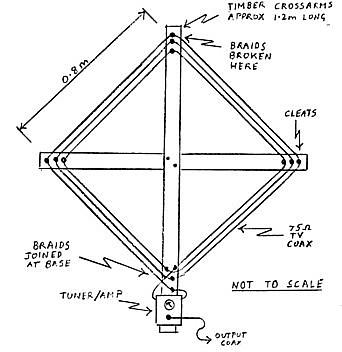Figure 1 - 3 Turn Coax Loop Aerial Circuit Diagram Figure 2 - 3 Turn Coax Loop Aerial Assembly

The increase in the number of turns of three to one does not fully compensate for the loss of 1:10 in area. However, the smaller three-turn loop measured a Q factor of 54 compared with 16 for the larger one-turn loop. The net result of all this was that loop sensitivity (Es/e) calculated as 3.9 for the smaller loop compared with 3.7 for the larger loop. Hence their performances could he expected to be much the same.

The natural resonant frequency of the three turn.loop was found to be around 3.5 MHz and well above the 1.8MHz operating frequency. It is possible that four turns could also have provided a natural resonance above 1.8 MHz, with a possible further improvement in sensitivity. However, this was not checked out.

Unshielded Loop Aerial

Theory on how a shielded loop aerial reduces localised noise interference was given in my earlier article on loop aerials for VLF-LF (Reference 1). If localised noise is not a problem, loop sensitivity can be improved by not shielding the loop.This reduces the loop self capacitance and hence the number of turns for a given upper frequency limit can be increased. I found that seven turns of lightgauge hook-up wire, spaced 5mm apart on the 0.8m square frame, produced a natural resonance of 2 MHz, just conveniently above the 1.8 MHz required. The Q factor at 1.8 MHz measured 39 and loop sensitivity calculated to a value of 6.5, which is very close to a value calculated for a 10m high vertical aerial.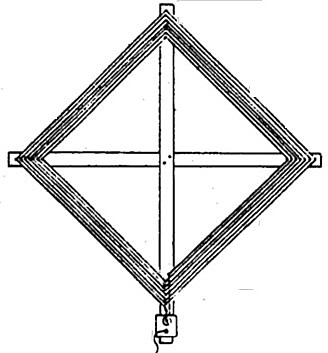Figure 3 Unshielded Loop Aerial

Conductor Size

As we have discussed earlier, the loop sensitivity at resonance is directly proportional to its Q factor which, in turn, is the ratio of its inductance to series resistance. The resistance is the sum of radiation resistance and the AC loss resistance in the loop, the latter being the prominent factor as the value of radiation resistance is very small. The AC loss resistance can be reduced by increasing the surface area of the loop conductor.

The original seven-turn unshielded loop was wound with 0.4mm diameter wire and produced a Q factor at 1.8 MHz of 39. The wire was ultimately replaced with a 1.7mm stranded conductor to improve the Q. A side effect in doing this was an increase in the selfcapacitance of the loop, making it barely possible to peak the loop tuning at 1.8 MHz. To correct for this, the inductance was reduced by reducing the number of turns to 6.5 or, more correctly, one of the seven turns was returned from halfway around the loop across one of the crossarms so that the one turn had half the area of the others. The larger diameter conductor increased the Q factor to around 100. It would have been higher had it not been limited by the 200 kOhm input resistance of the interface amplifier. Correcting for the reduced area of one turn and the increase in Q, the loop sensitivity (Es/e) was derived as a value of 15.6, considerably higher than the 6.5 derived for the 0.4mm conductor.

In all fairness to the original VK5KL large single-turn loop, I must point out that this was made with RG8 coax, which has an inner conductor diameter around 2.2min compared with the smaller diameter 0.8mm conductor in the coax used for my tests. The Q factor of the VK5KL loop might well have been much higher than I have quoted and hence its sensitivity greater. It also follows that I could have achieved higher sensitivity in my three turn coax loop had RG8 been used. However, it is assumed that relativity between the signal sensitivities of the two shielded loop forms tested would have been much the same had the larger cable been used in each..

Loop interface

To obtain the best advantage of the high Q factor of the loop (and hence its highest sensitivity) the loop is tuned to resonance at the operating frequency and connected via a high impedance input interface circuit. For the experiments described, this was achieved with the circuit shown in figure 4.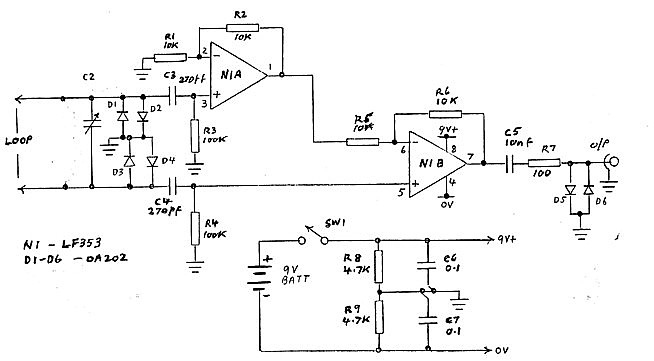Figure 4 Loop Tuner and Interface Amplifier
For 6 & 1/2 Turn Loop, C2 is 3-56 pf miniature variable Capacitor

The circuit makes use of twin JFET amplifier package type LF353 connected for balanced input. For the benefit of those who might not be quite familiar with operational amplifier theory, we will examine the stage gains. In the amplifier circuit around N1B, the gain via the inverting input is defined by the ratio R6/ R5 and since R5 and R6 are equal, the inverting gain is equal to -1. However, the gain via the non-inverting input is defined by the ratio R6/(R5+R6) and hence the non-inverting gain from the lower loop connection in the diagram is equal to 2.

The other loop connection is fed via the non-inverting input of N1A. As the circuit around N1A is identical to that around N1B, it also has a gain from the non-inverting input of 2. Since this connection of the loop is in anti-phase to the other connection, its signal via N1A must be inverted in mixing with the other signal in NlB. This is done via the inverting input of N1B without change of amplitude.

The loop aerial output is equally shared between the two amplifier inputs and hence the overall gain, balanced to unbalanced, is 2 or 6dB. This is about the limit one can get from the LF353 package at 1.8 MHz as its gain-bandwidth product is 4 MHz.

Tuning of the loop is set by variable capacitor C2 and, where necessary, parallel fixed capacitor Cl. The input circuit resistance is 200 kOhms, set by R3 and R4 in series. This is sufficiently high to prevent the loop Q from being lowered excessively.

The output resistance is largely set by R7, included for stability. Operational amplifiers can be very temperamental if operated directly into a capacitive load (such as a coaxial cable) without some series resistance.

The multitude of diodes at input and output are protection against excessive RF signal which might happen to be fed in. At the home installation, the loop aerial amplifier was connected via a switch into the receive side of the transceiver transmit/receive relay. This provided an interlock to prevent feeding the transmitter directly into the loop circuits. However, there was still a concern about RF induced from the transmitting aerial back into the loop and hence the diodes were included.

The amplifier circuit provides a very high impedance to low impedance conversion without loss of signal voltage developed by the loop Q.

Ferrite Core Loop Aerials

A further exercise was carried out to compare the performance of the loop aerial wound on a ferrite rod with that of the larger air-wound loops. Whilst this type of loop aerial has a very small loop area, the loss in area is compensated by the large number of turns which can be used and a high multiplying factor determined by the ferrite material permeability. For the aerial oriented to give maximum signal, the loop sensitivity formula is expanded to the following:

Es/e = (2nNAQu')/lambda

Where u'= The corrected permeability

Permeability requires some explanation. Permeability (u) of the material is the multiplying factor which applies to the inductance of the winding compared to when it is air wound, assuming all lines of magnetic flux pass through the winding. In the ferrite rod, not all lines of flux pass through the winding, so there is leakage flux. The inductance is therefore less, and a multiplying factor called rod permeability (ud) applies. Curves relating rod permeability to material permeability, for different rod length to diameter ratios, are published in the ARRL Antenna Handbook (reference 2) and in Amidon Associates brochures.

The corrected permeability (u') is the multiplying factor applied to the loop formula. If the coil winding is the full length of the ferrite rod, then corrected permeability is equal to rod permeability. If the rod is longer than the winding, the corrected permeability is increased as follows:

u' = urod x cube root(a/b)

where a = Length of the rod
b = Length of the winding

To carry out my tests, 1 purchased a ferrite rod (Cat L1401) from Dick Smith Electronics. The rod dimensions are 20cm. long by 9.5mm diameter. No information seemed to be available on permeability, hence the rod permeability was derived by calculating the ratio of inductance, measured for a given number of turns on the rod, to that for the same sized winding in air. The inductance in air was determined by two different methods which gave much the same answer. The first method was to apply the well-known Wheeler's formula for air-wound coils which can be found in many handbooks. The second method was to wind the same number of turns on a length of bamboo which happened to have the same diameter as the rod, and the inductance of this coil was then measured. The value of rod permeability was determined as 74, and from the curves previously mentioned, material permeability appeared to be around 120.

To operate at 1.8 MHz, 64 turns of 0.44mm single-core PVC-covered wire were wound around the ferrite rod. For this number of turns, the maximum which could be achieved, self-resonance was just above the 1.8MHz band at 2 MHz. The 64 turns occupied 7cm of the length of the rod and, from this measurement, a corrected permeability of 81 was derived.

The Q factor of the loop at 1.8 MHz was measured as 57, and loop sensitivity was calculated as 0.86, considerably less than all the air-wound loops discussed.

Comparison of Loop Sensitivities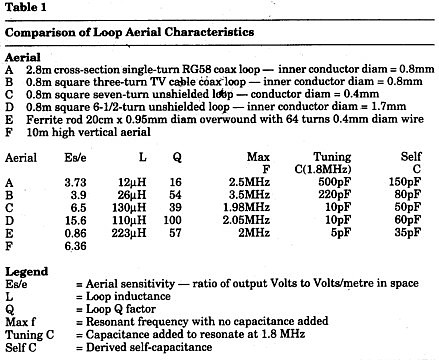The characteristics of the various loop aerials discussed are compared in Table 1. Despite its smaller area, the 0.8m square coax loop (B), with more turns and higher Q, has a signal sensitivity as good as the larger single-turn coax loop (A). With a self-resonance at 3.5 MHz, well above the required frequency of 1.8 MHz, it is probable that the sensitivity of (B) could have been improved further by adding another turn, still being tunable to 1.8 MHz.

The additional turns made possible by not shielding the seven-turn loop (C), enabled a higher signal sensitivity to be achieved comparable with that of a 10m vertical aerial (F). The importance of using a large sized conductor to reduce AC resistance is shown by comparing aerial (C) with aerial (D), which is similar to (C), but which has a large diameter conductor. The sensitivity of (D) is considerably higher than that of (C).

The ferrite rod loop aerial (E) works quite well because of the high permeability of its core, but it is no match in terms of signal sensitivity when compared with the larger air core loops.

Operational Performance

With the various loop aerials connected in turn to a receiver, the relative signal levels received followed much the same pattern as loop sensitivity shown in Table 1. Signal levels received on the three turn coax loop were comparable with those received on a sloping wire Marconi aerial loaded for 1.8 MHz and normally used as the transmitting aerial. The unshielded loops, with more turns, delivered considerably higher signal levels than the sloping wire. Some of the extra level is due to the 6dB gain in the interface amplifier but, even taking this into account, there was still quite a level difference.

Quite apart from the ability of the loop aerial to reduce interference from a localised noise source, its directional properties can be used to improve the signal-to-noise ratio in the presence of atmospheric noise. This particularly applies if the noise has a directional property and the loop is oriented so that its null position faces the direction of maximum noise. Of course, the same technique can be applied to a source of QRM. For these applications, the unshielded loops, with their higher signal sensitivities, seemed to work best and they clearly improved the readability of signals otherwise difficult to copy on the sloping wire.

One would expect that the coax loop aerial would be more suitable than the unshielded loop in an environment of high local noise. Notwithstanding this, the seven-turn unshielded loop did not appear to be any more sensitive to localised noise which was introduced in the radio shack.

In actual fact, the unshielded loops could be expected to have quite reasonable rejection of the electric field component when operated into the type of interface amplifier circuit used. The electric field component of localised noise is the one which is the highest level and this is induced into the loop in a common mode with equal voltage at the loop output terminals referred to ground. The amplifier has a differential input circuit and hence the electric field component is essentially balanced out (see footnote). If the balance is good, there would appear to be a lesser need for electrostatic shielding to reduce localised noise.The additional shield might be needed more in using the loop for accurate direction finding (DF) work where a small amount of pick-up as a vertical aerial (called vertical or antenna effect) could make an error in the position of the signal null.

The ferrite rod loop aerial has an advantage in its small size and suitability for portable applications. However, its performance when connected to a receiver did not match that of the 0.8m square loops.

Conclusions

The performance of 0.8m square loop aerials for 1.8 MHz has been discussed. It is concluded that a three or four-turn coax loop aerial of this size would work as well as the larger single-turn coax loop aerial. The discussion has also extended to experiments with the ferrite rod loop aerials. As stated earlier, the aerial has its limitations.

By using a loop of unshielded turns to reduce the capacitance, the number of turns and hence the loop sensitivity, can be increased. Provided that the unshielded loop aerial is operated in a balanced mode, rejection of localised noise is still quite good. Loop sensitivity is dependent on its Q factor, and to achieve a high Q, the conductor size, or at least its surface area, should be as large as practicable.

Provided that the loop circuit is well balanced, I see little point in shielding the loop unless accurate DF work is envisaged. Some texts describe a step down coupling transformer to interface the loop to the receiver input. As a preference, I favour the use of the high impedance amplifier for the following reasons: Firstly, the transformer reflects a load from the receiver input and this must lower the loop Q. Secondly, the transformer provides a high-to-low-impedance transfer with step down of voltage. The amplifier does this as a voltage follower, or even with voltage gain. The only precaution is that the amplifier must be selected for a noise level below that received expected from the loop. The higher the loop sensitivity, the less is the chance of this being a problem.

My recommendation for a good performance 1.8MHz loop aerial, small enough to operate both inside the radio shack or outside, is six or seven turns of a heavy gauge copper wire spaced 5mm to 10mm on a 0.8m square frame (See Figure 3). As an alternative to ordinary wire, one might consider connecting up the outer braid of the old style heavy shielded wire or some discarded coax cable. Connect via the interface amplifier of figure 4. Use an input tuning capacitor 3-56pf (miniature variable). The amplifier is mounted at the base of the frame to keep short connecting leads to the loop.

1. Lloyd Butler VK5BR - VLF-LF and the Loop Aerial. - Amateur Radio, August 1990.

2. C H Castle VK5KL - A l0 ft Diameter Receiving Loop Aerial on 1.8 MHz - Amateur Radio, March 1982.

3. The ARRL Antenna Handbook 15th Edition 1988 - Chapter 5, Loop Antennas, and Chapter 14, Direction Finding Antennas.

Footnote

I have pointed out in the text that when the loop is connected via the differential input circuit the electric field component is induced in a common mode against earth and is essentially balanced out by the circuit. This should be qualified as being conditional on the loop dimensions being small compared with a wavelength. If the plane of the loop is in line with the direction of signal, a phase difference must exist between the voltages induced into each side of the loop. This will develop a differential voltage between opposite sidewires of the loop. In the loop aerials discussed, the distance between the sides of the loop is 0.8m, small compared with a wavelength of 160m. Hence, the phase difference is small and the voltage generated is also assumed to be small.